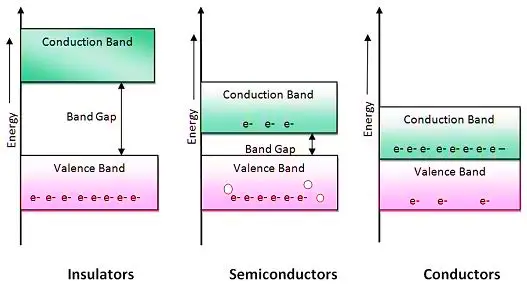# Material Classification based on Energy Band Diagram

## Band Gap Theory

The Band Gap theory explains the mechanism of conductor, insulators and semiconductor regarding the movement of free electrons from valence band to the conduction band.

An energy band which separates the conduction band and the valence band is called Band gap or Forbidden band or Forbidden gap.

Based on energy gap, the material is classified into three categories

1. Conductor
2. Insulator
3. Semiconductor

The fig. 1 shows the Classification of material based on energy band theory.Fig. 1 Classification of material based on energy band theory (e– = electrons and o = holes)

## 1. Conductor

In a conductor, there is a large number of free electrons at room temperature, so energy gap does not exist. The valence band and the conduction band overlap with each other.

Example: silver, copper, aluminium, gold, iron, steel, etc.

## 2. Insulator

In insulators, the energy gap is very high i.e. 7 eV (electron volt). So at a very high-temperature or voltage, electrons can not move from valence band to conduction band. So this material can not conduct at all.

Example: wood, paper, mica, plastic, etc.

## 3. Semiconductor

In a semiconductor, at absolute zero temperature, the conduction band is empty and it behaves like an insulator. At a normal temperature, some electrons move from valence band to conduction band. Hence, a semiconductor can conduct partially at normal or room temperature.

As temperature increases, energy gap decreases and a large number of free electrons are available.

Example: Silicon, germanium, etc.

• At room temperature, the energy gap of silicon and germanium is 1.12 eV and 0.78 eV, respectively.
• Semiconductors are the materials with the electric conductivity less than conductors (metals) and more than insulators.
error: Content is protected !!